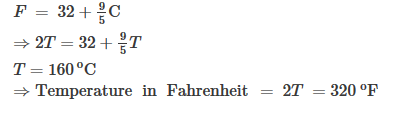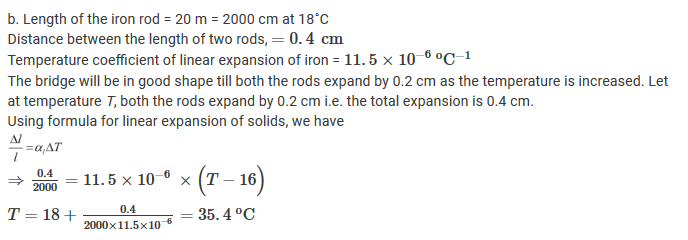☰ अधिक माहितीसाठी येथे क्लीक करा

Measurement and Effects of Heat

Question 1:

A. Whom should I Pair with?
 Group A Group B a. Temperature of a healthy human body 296 K b. Boiling point of water 98.6∘F c. Room temperature 0∘C d. Freezing point of water 212∘F

B. Who is telling the truth?
a. The temperature of a substance is measured in joules.
b.Heat flows from an object at higher temperature to an object at lower temperature.
c. Joule is the unit of heat.
d. Objects contract on heating.
e. Atoms of a solid are free.
f. The average kinetic energy of atoms in a hot objects is less than the average kinetic energy of atoms in a cold objects.

C. You will find if you search.
a. A thermometer is  used to measure............... .
b. The apparatus used to measure heat is called a..................... .
c. Temperature is the measures of the............................ Kinetic energy of the atoms in a substance.
d. The heat contained in a substannce is the measures of the....................... kinetic energy of atoms in the substance.

A.
 Group A Group B a. Temperature of a healthy human body 98.6∘F b. Boiling point of water 212∘F c. Room temperature 296 K d. Freezing point of water 0∘C

B.
a. Sentence a is lying as heat energy is measured in joules.
b. Sentence b is telling the truth as heat energy flows from an object at higher temperature to an object at lower temperature.
c. Sentence c is telling the truth as joule is the unit of heat.
d. Sentence d is lying as objects expand on heating.
e. Sentence e is lying as atoms of a solid are closely packed because of force attraction between them.
f.  Sentence f is lying as the average kinetic energy of atoms in a hot objects is greater than the average kinetic energy of atoms in a cold objects.

C.
a. A thermometer is  used to measure temperature.
b. The apparatus used to measure heat is called a calorimeter.
c. Temperature is the measures of the average kinetic energy of the atoms in a substance.
d. The heat contained in a substance is the measures of the total kinetic energy of atoms in the substance.

Question 2:

Nishigandha kept a vessel containing all the ingredients for making tea in a solar cooker. Shivani kept a similar vessel on a stove. Whose tea will be ready first and why?

Shivani's tea will be prepared first.
In Shivani's case, the intensity of the flame in contact with the vessel is very high due to which the flow of heat will be faster. Thus, the time taken by the tea to reach its boiling point will be less. Hence, tea will be prepared fast.
In case of Nishigandha, the intensity of radiation reaching the vessel is not as high as compared to stove's flame. Thus, the flow of heat will be slower in this case due to which more time will be taken to reach the boiling temperature of the tea. Hence, tea will be prepared at slower rate.

Question 3:

a. Describe a clinical thermometer. How does it differ from the thermometer used in laboratory?

b. What is the difference between heat and temperature ? what are their units.

c. Explain the construction of a calorimeter. Draw the necessary figure.

d. Explain why rails have gaps at specific distances.

e. Explain with the help of formulae the expansion coefficients of liquid and gas.

a. Clinical thermometer is used in homes. It has a glass tube with a bulb at one end and the other end closed. This bulb and some part of the tube is filled with thermometric liquid, such as mercury or alcohol. The rest of the volume of the tube has vacuum in it. There is a kink near the end of the glass tube which prevents the falling back of the thermometric liquid once it is removed from the hot body under observation.
It is basically used to measure the temperature of humans. A clinical thermometer has a temperature range of only 35 ºC to 42 °C.b.
 Clinical thermometer Laboratory thermometer This thermometer is used in homes. It is basically used to measure the temperature of human body. This thermometer is used to measure the temperature of all things, except the human body. A clinical thermometer has a temperature range of only 35 ºC to 42 °C. A laboratory thermometer has a temperature range of -10 ºC to 110 ºC. It has kink. It does not have kink.b.
 Heat Temperature It is a form of energy which causes in us the sensation of hotness or coldness. It is measure of the degree of hotness or coldness of an object. It is measured in joules (J). It is measure in kelvin (K), Celsius (oC) and Fahrenheit (oF)

c. Calorimeter A device used for heat measurement is called a calorimeter.
Construction of a Calorimeter
• It consists of a metallic vessel and stirrers. They are made of copper or aluminium.
• The vessel is then kept inside a wooden jacket which contains heat-insulating materials.
• The outer wooden jacket acts as a heat shield, and reduces the heat loss from the inner vessel.
• The outer jacket has an opening through which a mercury thermometer is inserted into the calorimeter.d. All solids expand on heating. As rails are made up of steel, these also expand in summers and contract in winters. These expansion and contraction can cause sagging and bending of rails which could derail the trains running on them. Thus, the rails have been provided with gaps at specific distance so as to prevent this bending of rails. These spaces get closer in summers and wider in winters.

e. The formula for liquid expansion or gas is

From the above formula, we can say that
• the volumetric expansion coefficient of a liquid ($\beta$) is defined as the fractional change in the volume of the liquid per degree Celsius (or kelvin) change in temperature.
• the volumetric expansion coefficient of a liquid ($\beta$) is defined as the fractional change in the volume of the gas per degree Celcius (or kelvin) change in temperature at constant pressure. So, $\beta$ is the constant pressure volumetric expansion coefficient in case of gas.

Question 4:

Solve the following examples.

a.What must be the temperature in Fahrenheit so that it will be twice its value in Celsius?

b. A bridge is made from 20 m long iron rods. At temperature 18C, the distance between two rods in 0.4 cm. Up to what temperature will   the bridge be in good shape?

c. At 15C the height of Eifel tower is 324 m. If it is made of iron, what will be the increase in length in cm, at 30C?

d. Two substances A and B have specific heats c and 2 c respectively. If A and B are given Q and 4Q amounts of heat respectively, the change  in their temperatures is the same. If the mass of A is m, what is the mass of B?

e. When a substance having mass 3 kg receives 600 cal of heat, its temperature increases by 10C. What is the specific heat of the substance?

$\mathrm{}$$\mathrm{}$Reviewed by Amol Uge on January 05, 2019 Rating: 5